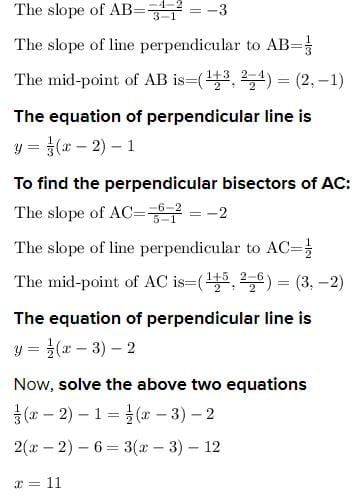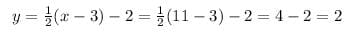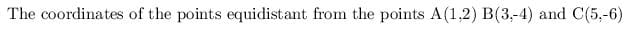Test: Coordinate Geometry- 2

# Test: Coordinate Geometry- 2

Test Description

## 10 Questions MCQ Test Quantitative Aptitude (Quant) | Test: Coordinate Geometry- 2

Test: Coordinate Geometry- 2 for Quant 2023 is part of Quantitative Aptitude (Quant) preparation. The Test: Coordinate Geometry- 2 questions and answers have been prepared according to the Quant exam syllabus.The Test: Coordinate Geometry- 2 MCQs are made for Quant 2023 Exam. Find important definitions, questions, notes, meanings, examples, exercises, MCQs and online tests for Test: Coordinate Geometry- 2 below.
Solutions of Test: Coordinate Geometry- 2 questions in English are available as part of our Quantitative Aptitude (Quant) for Quant & Test: Coordinate Geometry- 2 solutions in Hindi for Quantitative Aptitude (Quant) course. Download more important topics, notes, lectures and mock test series for Quant Exam by signing up for free. Attempt Test: Coordinate Geometry- 2 | 10 questions in 10 minutes | Mock test for Quant preparation | Free important questions MCQ to study Quantitative Aptitude (Quant) for Quant Exam | Download free PDF with solutions
 1 Crore+ students have signed up on EduRev. Have you?
Test: Coordinate Geometry- 2 - Question 1

### Distance of a point from the origin: The distance of a point A(x, y) from the origin O(0, 0) is given by OA = √x2 + y2 Find the distance of the point A(4, -2) from the origin.

Detailed Solution for Test: Coordinate Geometry- 2 - Question 1

OA = √4 - 02+(-2 - 0)2 = √16+4 = √20 = radic;4*5 = 2√5 units

Test: Coordinate Geometry- 2 - Question 2

### Distance between two points : If (x1, y1) and B(x2, y2) be two points, then AB = √(x2 - x1)2 + (y2 - y1)2 Find the distance between the points A(-4, 7) and B(2, -5).

Detailed Solution for Test: Coordinate Geometry- 2 - Question 2

AB = √(2+4)2 + (-5-7)2

= √62 + (-12)2

= √36+144 = √180

=√36*5 = 6√5 units.

Test: Coordinate Geometry- 2 - Question 3

### The distance between the points A(b, 0) and B(0, a) is.

Detailed Solution for Test: Coordinate Geometry- 2 - Question 3

AB = √(b-0)2-(0-a)2

= √b2+a2

= √a2+b2.

Test: Coordinate Geometry- 2 - Question 4

If the distance of the point P(x, y) from A(a, 0) is a + x, then y2 = ?

Detailed Solution for Test: Coordinate Geometry- 2 - Question 4

√(x-a)2+(y-0)2 = a + x

= (x-a)2+y2

= (a+x)2 => y2 = (x-a)2-(x-a)2-4ax => y2 = 4ax

Test: Coordinate Geometry- 2 - Question 5

The distance between the points A(5, -7) and B(2, 3) is:

Detailed Solution for Test: Coordinate Geometry- 2 - Question 5

AB2 = (2 - 5)2 + (3 + 7)2

=> (-3)2 + (10)2

=> 9 + 100 => √109

Test: Coordinate Geometry- 2 - Question 6

Area of a triangle :

If A(x1,y1), B(x2,y2 and C(x3, y3) be three vertices of a ΔABC, then its area is given by:

Δ = 1/2 [x1(y2 - y3 + x2(y3 - y1) + x3(y1 - y2)]

Find the area of ΔABC whose vertices are A(9, -5), B(3, 7) and (-2, 4).

Detailed Solution for Test: Coordinate Geometry- 2 - Question 6

Here, x1 = 9, x2 = 3, x3 = -2 and y1 = -5, y2 = 7, y3 = 4

= 1/2 [9(7-4) + 3(4+5) + (-2)(-5-7)]

= 1/2 [9(3) + 3(9) - 2(-12)]

= 1/2 [27 + 27 + 24]

= 1/2 

= 39 sq.units

Test: Coordinate Geometry- 2 - Question 7

Find the area of ΔABC whose vertices are A(2, -5), B(4, 9) and (6, -1).

Detailed Solution for Test: Coordinate Geometry- 2 - Question 7

Here, x1 = 2, x2 = 4, x3 = 6 and y1 = -5, y2 = 9, y3 = -1

= 1/2 [2(9+1) + 4(-1+5) + 6(-5-9)]

= 1/2 [2(10) + 4(4) + 6(-14)]

= 1/2 [20 + 16 - 84]

= 1/2 [-48]

= 24 sq.units

Test: Coordinate Geometry- 2 - Question 8

The points A(0, 6), B(-5, 3) and C(3, 1) are the vertices of a triangle which is ?

Detailed Solution for Test: Coordinate Geometry- 2 - Question 8

AB2= (-5 - 0)2 + (-3 - 0)2 = 25 + 9 = 34

BC2 = (3 + 5)2 + (1-3)2 = 82 + (-2)2 = 64 + 4 = 68

AC2 = (3 - 0)2 + (1 - 6)2 = 9 + 25 = 34.

AB = AC. ==> ΔABC is isosceles.

Test: Coordinate Geometry- 2 - Question 9

The points A(-4, 0), B(1, -4), and C(5, 1) are the vertices of

Detailed Solution for Test: Coordinate Geometry- 2 - Question 9

AB2 = (1 + 4)2 + (-4 - 0)2

= 25 + 16 = 41,

BC2 = (5 - 1)2 + (1 + 4)2 = 42 + 52

= 16 + 25 = 41

AC2 = (5 + 4)2 + (1 - 0)2

= 81 + 1 = 82

AB = BC and AB2 = BC2 = AC2

ΔABC is an isosceles right angled triangle

Test: Coordinate Geometry- 2 - Question 10

Find the coordinates of the point equidistant from the points A(1, 2), B (3, –4) and C(5, –6).

Detailed Solution for Test: Coordinate Geometry- 2 - Question 10

Given three points A(1,2) B(3,-4) and C(5,-6)

we have to find the coordinates of the point equidistant from the points.

The point that is equidistant from three points is called circumcenter which can be evaluated to find the perpendicular bisectors.

To find the perpendicular bisectors of AB:(11,2)

## Quantitative Aptitude (Quant)

163 videos|163 docs|131 tests
 Use Code STAYHOME200 and get INR 200 additional OFF Use Coupon Code
Information about Test: Coordinate Geometry- 2 Page
In this test you can find the Exam questions for Test: Coordinate Geometry- 2 solved & explained in the simplest way possible. Besides giving Questions and answers for Test: Coordinate Geometry- 2, EduRev gives you an ample number of Online tests for practice

## Quantitative Aptitude (Quant)

163 videos|163 docs|131 tests